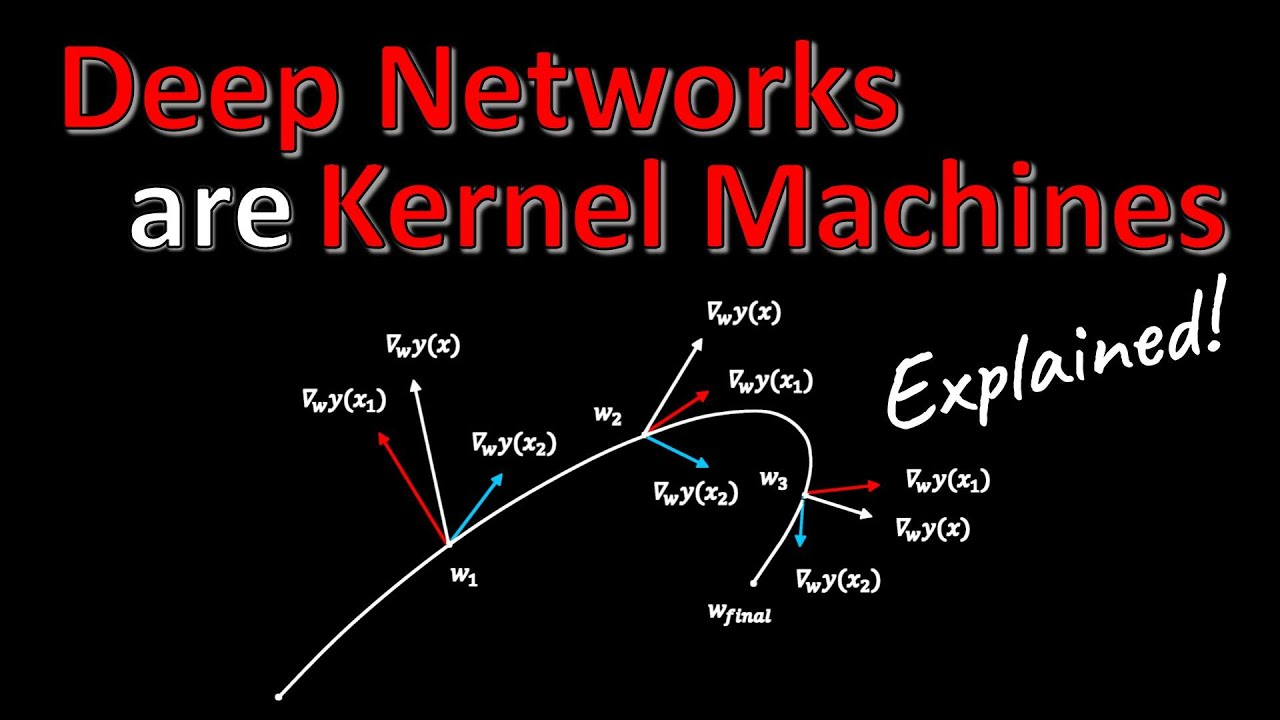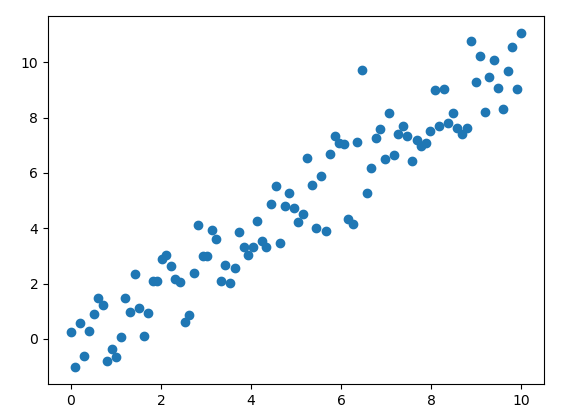Linear RegressionAI Deep Learning

Deep Networks Are Kernel Machines

Yannic Kilcher explains the paper “Every Model Learned by Gradient Descent Is Approximately a Kernel Machine.” Deep Neural Networks are often said to discover useful representations of the data. However, this paper challenges this prevailing view and suggest that rather than representing the data, deep neural networks store superpositions of the training data in their […]Machine Learning Statistics

Principal Component Analysis (PCA) Explained

Principal component analysis (PCA) is a workhorse algorithm in statistics, where dominant correlation patterns are extracted from high-dimensional data. Steve Brunton explains it in this great video.Data Science TensorFlow

Linear Regression: Concepts and Applications With TensorFlow 2.0

Linear regression is likely the first algorithm that you would learn when starting down a career path  in data science or AI, because it’s simple to implement and easy to apply in real-time. Here’s a great primer on how to do linear regression in TensorFlow 2.0. This algorithm is widely used in data science and […]Neural Networks

Neural Networks and Linear Regression

On Friday, someone asked me about linear regression with neural networks.  I didn’t have a good answer – I knew that you *could* do linear regression but neural networks, but never had actually done it in practice. Promising to learn more, I came across this video by giant_neural_network on YouTube.Machine Learning

Stanford Machine Learning Lecture on Linear Classifiers and SGD

Professor Percy Liang, Associate Professor of Computer Science and Statistics, delivers a lecture on Linear Classifiers and SGD.Data Science Machine Learning

What Can NFL Team Loyalties Teach Us About Linear Regression

In this LinkedIn Live session, I noticed an interesting pattern of NFL team merchandise that acted as an indicator of where in the state was more “Baltimore” or more “DC.”AI Python

Use ML.NET in Python to Create a Linear Regression Model with NimbusML

Jon Wood demonstrates how to use ML.NET within Python by using the NimbusML package. This video also gives an example of creating a linear regression model. Notebook – https://github.com/jwood803/MLNetExamples/blob/master/MLNetExamples/Notebooks/NimbusML/Regression.ipynb NimbusML documentation – https://docs.microsoft.com/en-us/nimbusml/overview